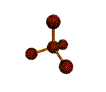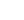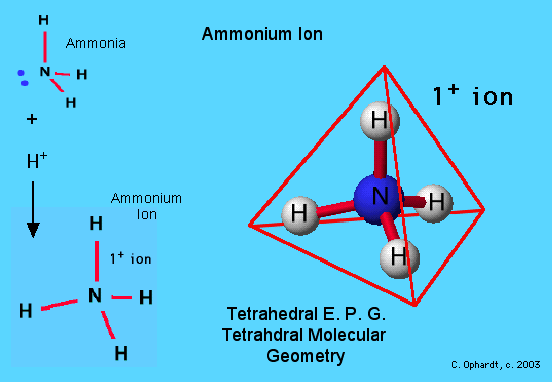# Tetrahedral Molecular Geometry

This molecule is made up of 4 equally spaced $$sp^3$$ hybrid orbitals forming bond angles of 109.5o. The shape of the orbitals is tetrahedral. Since there is an atom at the end of each orbital, the shape of the molecule is also tetrahedral.AX4Shape: tetrahedral Steric Number: 4 Lone Pairs: 0 Polar/NonPolar: NonPolar Hybridization: sp3 Examples: CH4, SiH4

## Methane

An example of tetrahedral electron pair geometry and molecular geometry is $$CH_4$$. The carbon has 4 valence electrons and thus needs 4 more electrons from four hydrogen atoms to complete its octet. The hydrogen atoms are as far apart as possible at 109o bond angle. This is tetrahedral geometry. The molecule is three dimensional. Methane is the simplest hydrocarbon molecule present in natural gas. This molecule provides the basis for the tetrahedral geometries at each carbon in a hydrocarbon chain.## Ammonium Ion

In this example, NH4+, the Lewis diagram shows N at the center with no lone electron pairs. Contrast this with ammonia, NH3, which does have a lone pair. The fourth hydrogen bonds to the ammonia molecule as a hydrogen ion (no electrons) bonding to the lone pair on the nitrogen.

This shows tetrahedral geometry for both the electron pair geometry and the molecular geometry. Ammonium ions are found in solutions of household ammonia or window cleaner. It is also found as part of a solid as ammonium nitrate in fertilizer.## Phosphate Ion

In this example, PO43-, the Lewis diagram shows phosphorus at the center with no lone electron pairs. The phosphorus and and one oxygen are bonded through a double bond which counts as "one electron pair". Hence the molecule has four electron pairs and is tetrahedral.

The Lewis diagram is as follows:
P = 5 e-
O = 6e- x 4 = 24e-
3- charge = 3e-
Total electrons = 32

Phosphorus is an atom that may exceed an octet of electrons. Phosphates are found in certain types of rocks, which are mined and converted to fertilizer. Plants need phosphate to grow as it is incorporated in the DNA and RNA genetic molecules. Furthermore phosphate is an important structural component of bones and teeth.